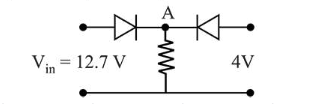# Both the diodes used in the circuit shown are assumed to be idealQuestion:

Both the diodes used in the circuit shown are assumed to be ideal and have negligible resistance when these are forward biased. Built in potential in each diode is $0.7 \mathrm{~V}$. For the input voltages shown in the figure, the voltage (in Volts) at point $\mathrm{A}$ is________Solution:

(12) Right hand diode is reversed biased and left hand diode is forward biased.

Hence Voltage at 'A'

$V_{A}=12.7-0.7=12 \mathrm{volt}$